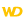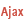• Detailed format of the character string to obtain
• Limitations
• Convert a size in bytes
WINDEVWEBDEVWINDEV MobileOthersNumToString (Function)
In french: NumériqueVersChaîne
Converts a numeric value (integer, real or currency) to a string according to a specified format.In this version, the numeric value to convert must be an integer or real.
Example
NumToString(123) // Returns "123"
NumToString(1.23) // Returns "1.23"
NumToString(12345.5, "+10.2f") // Returns " +12345.50"
NumToString(12345.5, "-+10.2f") // Returns "+12345.50 "
NumToString(12345.5, "+010.2f") // Returns "+012345.50"
NumToString(12345.5, "010.2f") // Returns "0012345.50"

NumToString(12345.5, "10.2fS")
// Returns " 12 345.50" (the thousand separator is " ")

NumToString(12345.5, "10,2fS")
// Returns " 12 345,50" (the decimal separator is ",")
Syntax
<Result> = NumToString(<Number> [, <Format>])
<Result>: Character string
String representing the number according to a specified format.
If <Number> is a string, the returned string is the same as the initial string. The format is ignored.If <Number> is a string, the string is converted as if it were a number.
<Number>: Integer or real
Numeric value to convert to a string (according to the specified format).
<Format>: Optional character string or constant
• String format expressed as follows:
"[<Align>][<Sign>][<Zero>][<Length>][<.Precision>]<Type>[<Thousand>]"The string is expressed in the following format:
"[<Zero>][<Align>][<Length>][<.Precision>]<Type>"

For more details, see the remarks.
•Constant used to define a system format:
Remarks

Detailed format of the character string to obtain

To convert a numeric value to a string, you need to specify the format of the string to be obtained. The format is as follows:
"[<Align>][<Sign>][<Zero>][<Length>][<.Precision>]<Type>[<Thousand>]"The format is as follows:
"[<Zero>][<Align>][<Length>][<.Precision>]<Type>"
The possible values are:
 If this parameter is specified, forces the number to be left-aligned ( = "-"). By default, the number is right-aligned. If this parameter is specified, displays a sign for positive numbers ( = "+"). This sign will appear to the left of the first significant digit. By default, only negative numbers have a "-" sign to the left of the first significant digit.Special case: if this parameter is specified and the number is equal to 0, the "+" sign will be displayed. This sign will also be displayed if a negative number becomes 0. If this parameter is specified ( ="0"), the number will be padded with leading zeros if the first significant part of the number is smaller that the specified length and the number is right-aligned. By default, the number is not padded with leading zeros "0".For example: NumToString(123, "05d") will return "00123". Defines the total length of the result string. The total length corresponds to the following elements: sign, thousand separator, decimal point, exponent, integer and decimal parts of the number. The length must be less than 100. If the specified length is too small to contain the significant part of the number, it will be ignored. By default, the number will be the same length of the significant part (it cannot be padded with zeros). This is also the case if = 0. If the specified length is greater than the significant part of the number:the result string will be padded with trailing spaces if the number is left-aligned, the result string will be padded with leading spaces or zeros if the number is right-aligned.Defines the total length of the result string or the total length of the integer part if the number is padded with zeros. The total length corresponds to the following elements: sign, thousand separator, decimal point, exponent, integer and decimal parts of the number. If the specified length is too small to contain the significant part of the number, it will be ignored. By default, the number will be the same length of the significant part (it cannot be padded with zeros). This is also the case if = 0. If the specified length is greater than the significant part of the number:the result string will be padded with trailing spaces if the number is left-aligned, the result string will be padded with leading spaces or zeros if the number is right-aligned. <.Precision> Defines the decimal separator ("." or ",") and the number of decimal places (for float ("f") and exponent ("e") only). Decimal separator: If the decimal separator is a comma, the decimal separator used will be the one defined: in the linguistic parameters of Windows.in the Windows configuration of the server.for the current user. If the decimal separator is a period, the decimal separator used will be a period. Remarks: ChangeSeparator has no effect on this separator. This function affects the display mask of numeric values in the controls.The <,Precision> syntax cannot be used: you must use <.Precision>. Number of decimals: The number of decimal places must be less than 100. If the number of decimal places in the number is greater than , the decimal part is rounded to the specified number of decimal places.If the number of decimal places in the number is less than , the decimal part will be padded with trailing zeros "0".The value of can be greater than . In this case, is ignored.Defines the number of decimals (for the float ("f") and exponent ("e") only). If the number of decimal places in the number is greater than , the decimal part is rounded to the specified number of decimal places.If the number of decimal places in the number is less than , the decimal part will be padded with trailing zeros "0".The value of can be greater than . In this case, is ignored if the number is padded with spaces. Type of result, mandatory parameter: d: Integer: A real number will be rounded to the nearest integer.e: Exponential notation:If is not specified, the default precision is 6.f: Decimal notation:If is not specified, the default precision is 6.x and X: Hexadecimal notation (X for uppercase letters):Only the integer part of the number is taken into account (no rounding).Special case:1. Length not specified and negative number: 20 hexadecimal characters for a currency, 8 characters for all the other numeric types.2. Negative number: the length of the resulting string will be at least the length that allows the sign bit to be specified. For example: -32767 if the "3X" format will return "8001"o: Octal notation: Only the integer part of the number is taken into account (no rounding). Special case:1. Length not specified and negative number: 27 hexadecimal characters for a currency, 11 characters for all the other numeric types.2. Negative number: the length of the resulting string will be at least the length that allows the sign bit to be specified. For example: -32767 in the "3o" format will return "700001" Thousands separator ( = "S" or "s"). The digits in the integer part of the number will be grouped by 3 and separated by the thousands separator defined in Windows. The sign (if any) will always be displayed to the left of the first significant digit. There should be no thousands separators between the sign and the first digit.The thousands separator is always a space (' ').Limitations

• The "f" format returns a valid result for numbers between -1e+17 and 1e+17.
• "x", "d" or "o" formats: the integer part must not exceed 99 999 999 999 999 999.
•How to use a comma for the decimal separator?
If the Windows configuration on the server does not use a comma as a decimal separator, you can:
• use a numeric Edit control (hidden if necessary). The displayed value can be obtained with DisplayedValue.
• use a formatted display control.

Convert a size in bytes

To convert a size in bytes, use LengthToString.
Business / UI classification: Neutral code
Component: wd280std.dll
Minimum version required
• Version 9